# Correlation is Not (Always) Transitive￼

##### Background

At first, I found this really puzzling.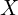is correlated (Pearson) with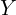, andis correlated with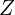. Does this meanis necessarily correlated with? Intuitively, this totally makes sense. The answer, however, is “no.”

Perhaps the strangest thing is how easy it is to rationalize this “puzzle.” I drink more beer () and read more books () when I am on a vacation (). That is, both pairs –andandand– are positively correlated. But I do not drink more beer when I read more books –andare not correlated. It is now obvious that correlation is not (always) transitive, but a second ago, this sounded bizarre.

Let’s go through the math.

##### Digging a Bit Deeper

Let’s denote the respective correlations between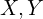andby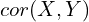,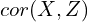, and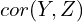. For simplicity (and without loss of generality), let’s work with standardized versions of these variables – that is, means of 0 and variances of 1. This implies,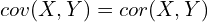for any pair.

We can write the linear projections of X and Z on Y as follows: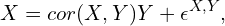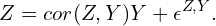Then, we have: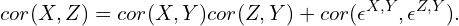We can use the Cauchy-Schwarz inequality to bound the last term, which gives the final range of possible values for: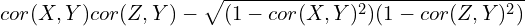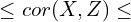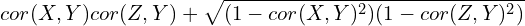For instance, if we set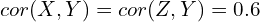, then we get: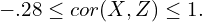That is,can be negative.

##### An Extremely Simple Example

Perhaps the simplest example to illustrate this is:

•andare independent random variables,
•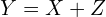.

The result follows.

The following code sets up this example in R.

set.seed(68493)
x <- runif(n=1000)
z <- runif(n=1000)
y <- x + z

Below is a table with correlation coefficients and p-values associated with the null hypotheses that they are equal to zero.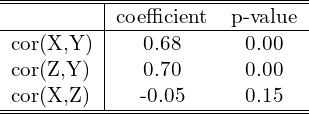You can find the code for this exercise in this GitHub repository.

##### When Is Correlation Transitive

From the equation above it follows that when bothand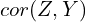are sufficiently large, thenis sure to be positive (i.e., bounded below by 0).

In the example above, if we fix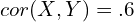, then we need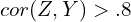to guarantee that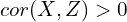.